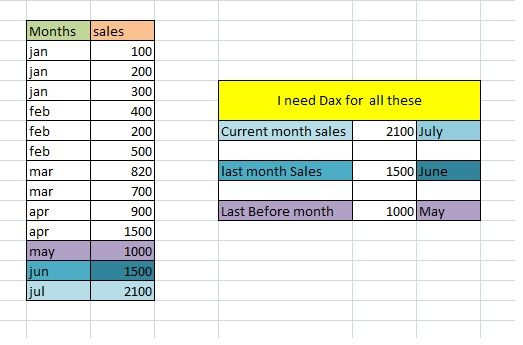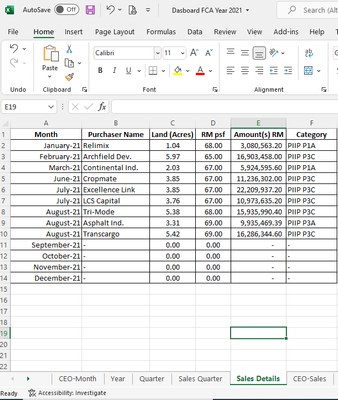cancel
Showing results for
Did you mean:Helper III

## Current Month , Previous Month and Before Previous Month

HI  PBI Experts ,

Here is my question for you in dax ,

i want to know the sales of the "current month", "last month", "last before month sales"  by using the Dax.

i am using previousmonth dax function  and i want to know for  current month  , before previous month dax function.will give kudos

Thanks

sandeep

1 ACCEPTED SOLUTIONResident Rockstar

If you only want to calculate the sales of "current month", "last month", "last before month sales"  by using the Dax, you could try the formula below.

```current_month_sales = CALCULATE(SUM('Table1'[Sales]),FILTER('Table1',MONTH('Table1'[Month])=MONTH(TODAY())))

last_month_sales = var current_month= MONTH(TODAY()) return  CALCULATE(SUM('Table1'[Sales]),FILTER('Table1',MONTH('Table1'[Month])=current_month -1))

last before_month_sales = var current_month= MONTH(TODAY()) return  CALCULATE(SUM('Table1'[Sales]),FILTER('Table1',MONTH('Table1'[Month])=current_month -2))

```

Here is the output.Best Regards,

Cherry

Community Support Team _ Cherry Gao
If this post helps, then please consider Accept it as the solution to help the other members find it more quickly.
27 REPLIES 27Frequent Visitor

this is a very good example for such cases... I have implemented it and using it...Anonymous
Not applicable
CALCULATE(SUM('Orders Combined'[LDS]),
FILTER('Calendar',MONTH('Calendar'[Date]) = MONTH(TODAY())-1),
FILTER('Calendar',YEAR('Calendar'[Date]) = IF(MONTH(TODAY())= 1,YEAR(TODAY())-1,YEAR(TODAY()))))

This may be another optionRegular Visitor

This works....

• Current Month Value = CALCULATE(SUM(Table1[Value]),MONTH(Table1[Originated])=MONTH(TODAY()))

This does not... Know why is does this?

• previous month = var current_month = MONTH(TODAY())
return CALCULATE(SUM('Table1'[Value]),FILTER('Table1',MONTH('Table1'[Originated])=current_month-1))Resident Rockstar

If you only want to calculate the sales of "current month", "last month", "last before month sales"  by using the Dax, you could try the formula below.

```current_month_sales = CALCULATE(SUM('Table1'[Sales]),FILTER('Table1',MONTH('Table1'[Month])=MONTH(TODAY())))

last_month_sales = var current_month= MONTH(TODAY()) return  CALCULATE(SUM('Table1'[Sales]),FILTER('Table1',MONTH('Table1'[Month])=current_month -1))

last before_month_sales = var current_month= MONTH(TODAY()) return  CALCULATE(SUM('Table1'[Sales]),FILTER('Table1',MONTH('Table1'[Month])=current_month -2))

```

Here is the output.Best Regards,

Cherry

Community Support Team _ Cherry Gao
If this post helps, then please consider Accept it as the solution to help the other members find it more quickly.Thanks a lot, this works like magic. Though I need a small help further. I have more than 1 year's data in the query. So the current_month-1 picks all months that are falling into this. E.g. Month(Today()) returns 9 for September, then the formula picks "8" which is August of 2022, 2021 both in my case. Any ideas on how to tackle this?

Thanks

SangeetaNew Member

-- FILTER('Data',YEAR(Data[Date Created])=current_year))Responsive ResidentFrequent Visitor

Thanks guys,

I have a similar issue. How I can accomplish the cumulative SUM of ALL previous months and to dynamically show this SUM as of current month?

` `Frequent Visitor

will this work if the month is january ? (with several decembers for each year in the data)Regular Visitor

Hi, would like to ask what is the right syntax if you have a slicer for date, and the analysis will automatically show output based on the date/month selected?

Example if you choose april 2022 in the slicer and then the output should be March 2022 (last month) and Feb 2022 (last 2 months)

thank you!Helper IV

Right, for me and what I was trying to do was to identify with a 1-Yes, 0-No what the is current month on my calendar table and MONTH(TODAY() -1 was not taking into account the year when rolling into the new year so I used the below.

IF(
STARTOFMONTH('DimCalendar'[Date]) = DATE(YEAR(TODAY()), MONTH(TODAY()),1),
"Yes",
"No"
)New Member

Hi.. Can everyone help me how i can do powerbi for previous month based on data given.

Thank you.Helper IV

Is your Month field a date and this is the formatting (January-21)?  If so you can reference that field using the PREVIOUSMONTH(DateTime'[DateKey]) if you just want the previous month date.  If you are looking to get the "Amount(s) RM" from previous month then use = CALCULATE(SUM(yourtablename[Amount(s) RM]), PREVIOUSMONTH(yourtablename[Month]))New Member

Hi Fo88er,

what do means youtablename ... Sales Detail ?Thanks.Helper IV

How do you handle last month sales for Dec 2021 when we are in Jan 2022? I am trying to find a good is last month logic that take into account when we roll into a new year. The below works if in current year (multiple years of dates in the dataset)

IsPreviousMonth =
IF(
YEAR ('Fact1Staff Consolidated GP - No Hours'[1Staff Transaction Date.TRX Dates]) = YEAR ( TODAY () )
&& MONTH ('Fact1Staff Consolidated GP - No Hours'[1Staff Transaction Date.TRX Dates]) = MONTH ( TODAY () ) -1,
1,
0
)Regular Visitor

I have the same problem as you mentioned with the new year.
It's not clear how you wanna integrate:

IsPreviousMonth =
IF(
YEAR ('Fact1Staff Consolidated GP - No Hours'[1Staff Transaction Date.TRX Dates]) = YEAR ( TODAY () )
&& MONTH ('Fact1Staff Consolidated GP - No Hours'[1Staff Transaction Date.TRX Dates]) = MONTH ( TODAY () ) -1,
1,
0
)
Do you finally found a good solution ?Helper IV

Yes, I used this

IF(
STARTOFMONTH('DimCalendar'[Date]) = DATE(YEAR(TODAY()), MONTH(TODAY()),1),
"Yes",
"No"
)Regular Visitor

Try this:

``````Measure =
VAR _index = 0
VAR _StartMonth = DATE( YEAR( TODAY() ), MONTH( TODAY() ) - _index , 1)
VAR _StartNextMonth = EOMONTH( _StartMonth, 0) + 1
VAR _Amount = CALCULATE( [Sales], Calendar[Date] >= _StartMonth, Calendar[Date] < _StartNextMonth)
RETURN _Amount``````

For each of the three measures you are trying to make, you just change the _index:

• Current Month: _index = 0
• Last Month: _index = 1
• Last Before Month: _index = 2

and so on... You can apply for how many months before or after you want. Hope I helped.

Seya!Regular Visitor

I cannot understand why the LM value does not appear in the yellow cell when I apply the external filter. Can anyone help me? I already appreciate any help please.

I enclose the data file for you to analyze.Anonymous
Not applicable

Thank you so much, this helped me solve my issue as well!